# 4. Suppose your bicycle tire is fully inflated, with an absolute pressure of 7.00 x 10°...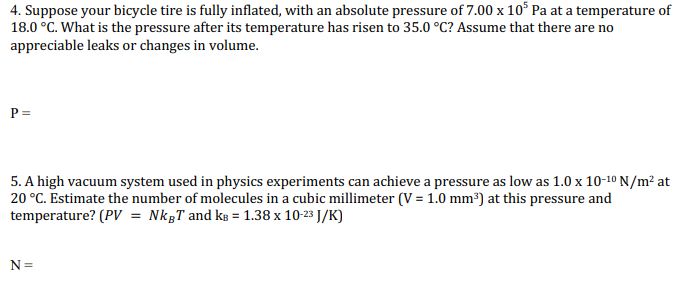4. Suppose your bicycle tire is fully inflated, with an absolute pressure of 7.00 x 10° Pa at a temperature of 18.0 °C. What is the pressure after its temperature has risen to 35.0 °C? Assume that there are no appreciable leaks or changes in volume. PE 5. A high vacuum system used in physics experiments can achieve a pressure as low as 1.0 x 10-10 N/m² at 20 °C. Estimate the number of molecules in a cubic millimeter (V = 1.0 mm3) at this pressure and temperature? (PV = NkgT and kb = 1.38 x 10-23 J/K) N =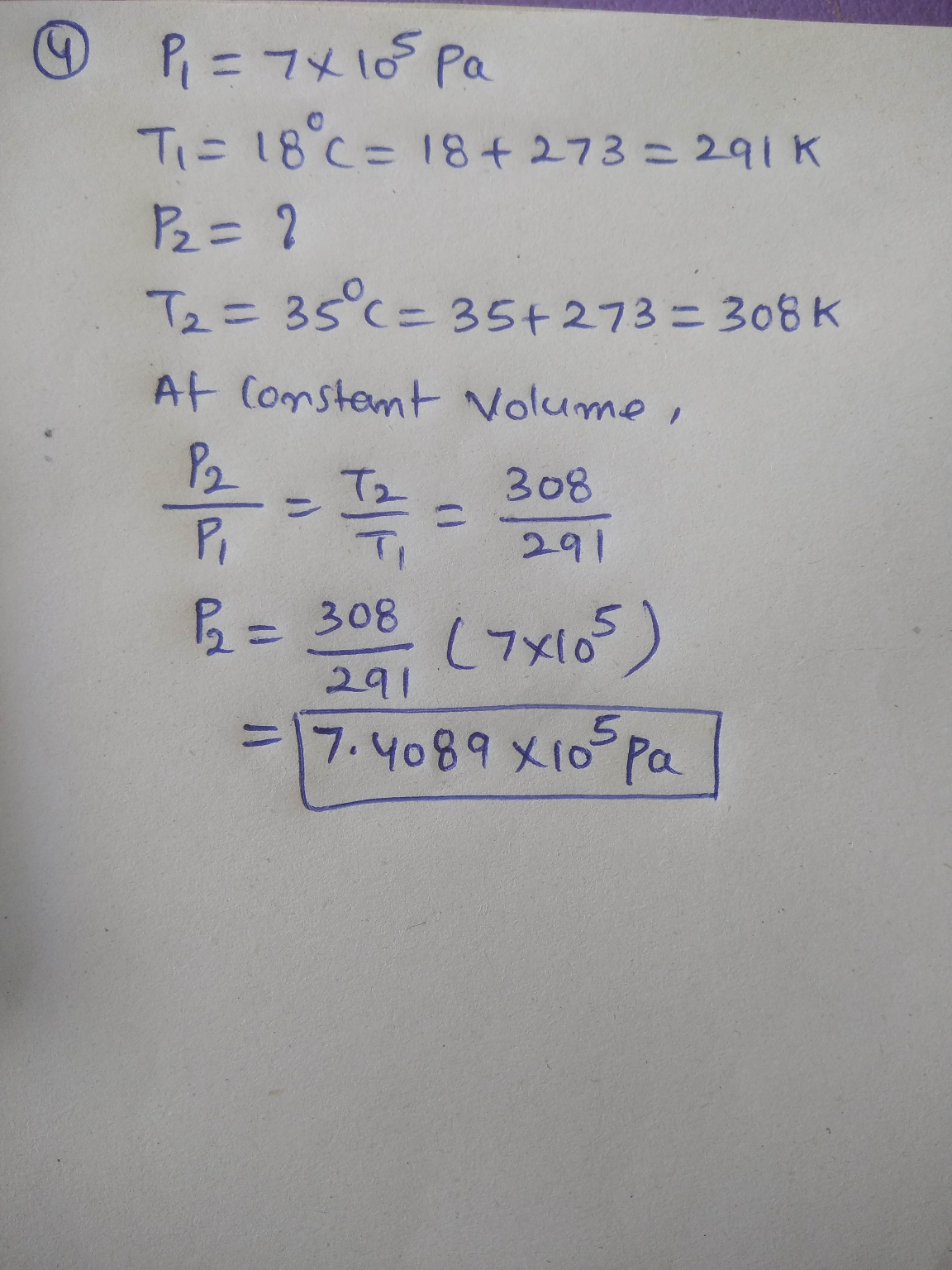SOLUTION :

4.

P/T = constant for the given volume.

=> P1/T1 = P2/T2

=> P2 = P1 (T2/T1) = 7 * 10^5 ((273+35) / (273+18))

=> P2 = 740893.47  Pa

So, pressure at 35ºC will be 740893.47 Pa (ANSWER).

5.

PV = N Kb T

=> 1*10^(-10) * 1.0 * 10^(-9)  = N * 1.38*10^(-23) * (273+20)

=> 1 * 10^(-19) = 4.0434*10^(-21) * N

=> N =  1 * 10^(-19) / (4.0434 * 10^(-21)) = 24.73 molecules

So, 24.73 molecules are there in 1 cubic mm volume. (ANSWER).

#### Earn Coin

Coins can be redeemed for fabulous gifts.

Similar Homework Help Questions
• ### Suppose your bicycle tire is fully inflated, with an absolute pressure of 6.75 x 103 Pa...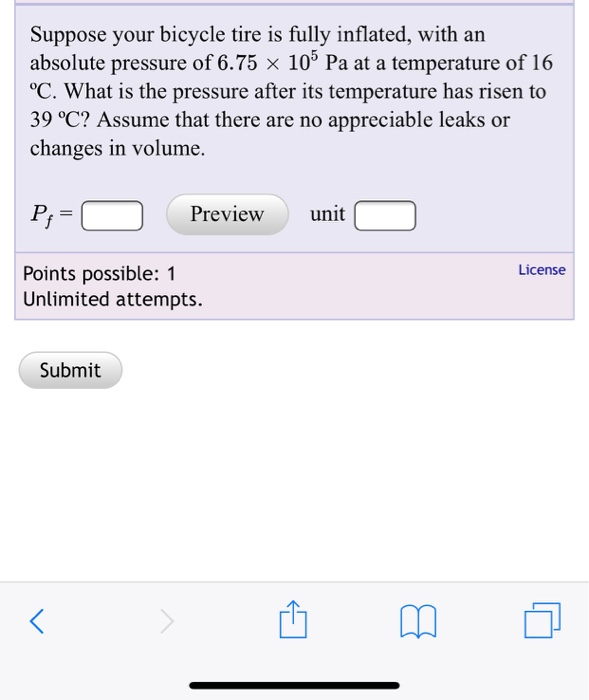Suppose your bicycle tire is fully inflated, with an absolute pressure of 6.75 x 103 Pa at a temperature of 16 °C. What is the pressure after its temperature has risen to 39 °C? Assume that there are no appreciable leaks or changes in volume. Preview unit License Points possible: 1 Unlimited attempts. Submit

• ### The ideal gas law states that PV = NkgT where P is the absolute pressure of...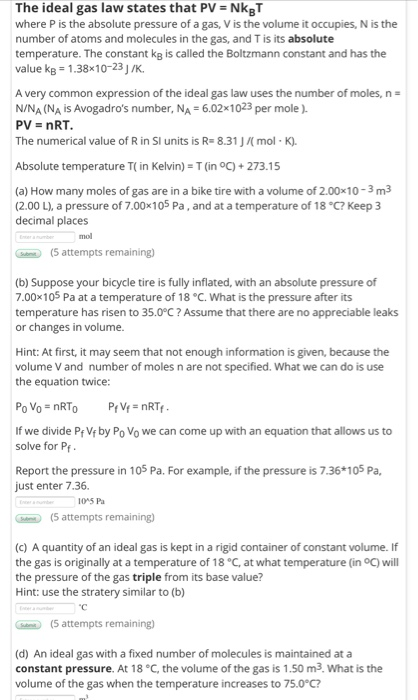The ideal gas law states that PV = NkgT where P is the absolute pressure of a gas, V is the volume it occupies, N is the number of atoms and molecules in the gas, and T is its absolute temperature. The constant ko is called the Boltzmann constant and has the value kg = 1.38x10-23J/K. A very common expression of the ideal gas law uses the number of moles, n- N/NA (NA is Avogadro's number, NA=6.021023 per mole). PV...

• ### 5. A steel-belted radial automobile tire is inflated to a gauge pressure of 2.10 x 10°...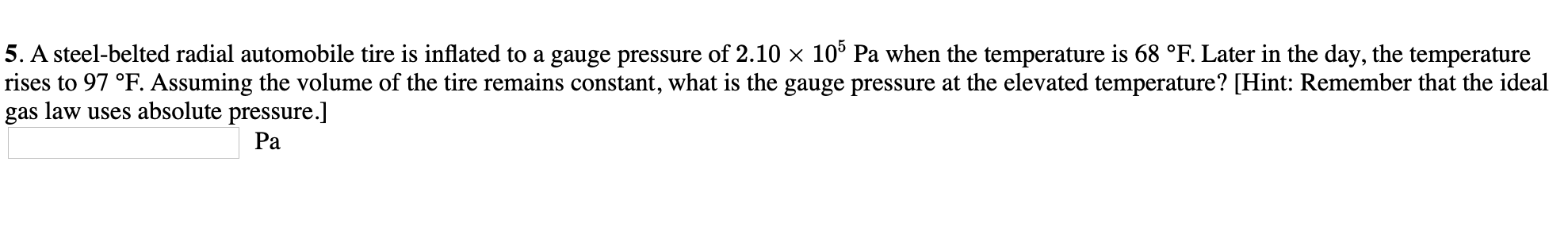5. A steel-belted radial automobile tire is inflated to a gauge pressure of 2.10 x 10° Pa when the temperature is 68 °F. Later in the day, the temperature rises to 97 °F. Assuming the volume of the tire remains constant, what is the gauge pressure at the elevated temperature? [Hint: Remember that the ideal gas law uses absolute pressure.] Ра

• ### 5. A steel-belted radial automobile tire is inflated to a gauge pressure of 1.85 x 10°...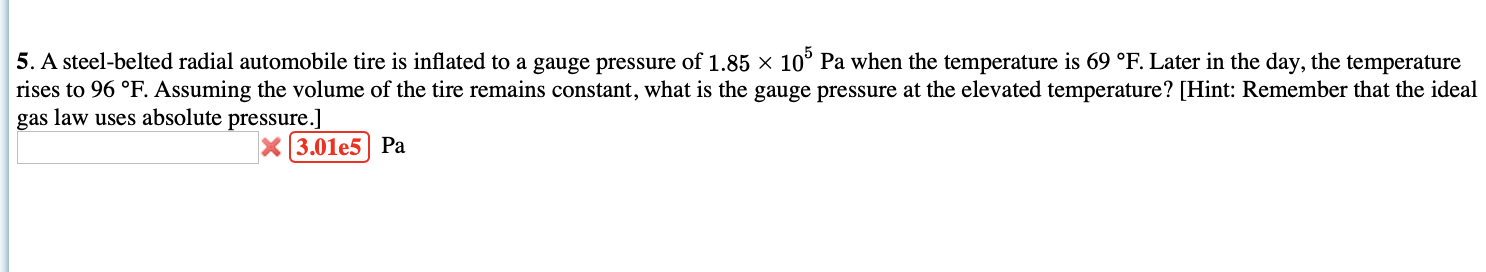5. A steel-belted radial automobile tire is inflated to a gauge pressure of 1.85 x 10° Pa when the temperature is 69 °F. Later in the day, the temperature rises to 96 °F. Assuming the volume of the tire remains constant, what is the gauge pressure at the elevated temperature? [Hint: Remember that the ideal gas law uses absolute pressure.] 3.01e5 Pa

• ### (9%) Problem 7: A bicycle tire has a pressure of 7.15 x 10 Pa at a...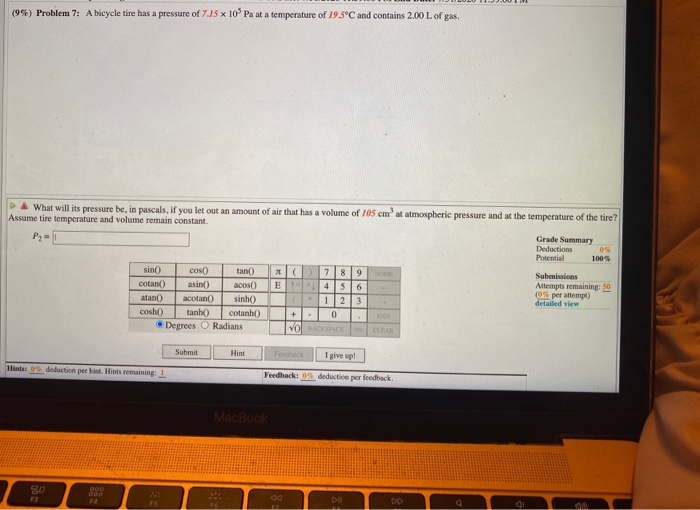(9%) Problem 7: A bicycle tire has a pressure of 7.15 x 10 Pa at a temperature of 19.5°C and contains 2.00 L of gas. What will its pressure be, in pascals, if you let out an amount of air that has a volume of 105 cm'at atmospheric pressure and at the temperature of the tire? Assume tire temperature and volume remain constant. Grade Summary Deductions 0% Potential 100% sino tan) 78 9 HOME Submissions cotan asino acos ETA 4...

• ### An expensive vacuum system can achieve a pressure as low as 1.00 x 10-8 N/m2 at...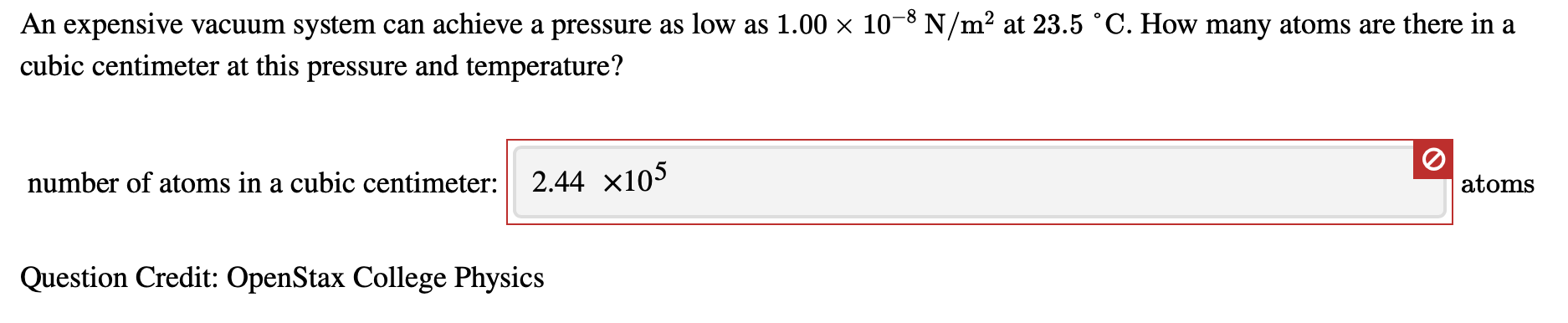An expensive vacuum system can achieve a pressure as low as 1.00 x 10-8 N/m2 at 23.5 °C. How many atoms are there in a cubic centimeter at this pressure and temperature? number of atoms in a cubic centimeter: 2.44 X10 atoms Question Credit: OpenStax College Physics

• ### Please answer all parts of the question: a,b,c,d Ideal Gas Law The ideal gas law states...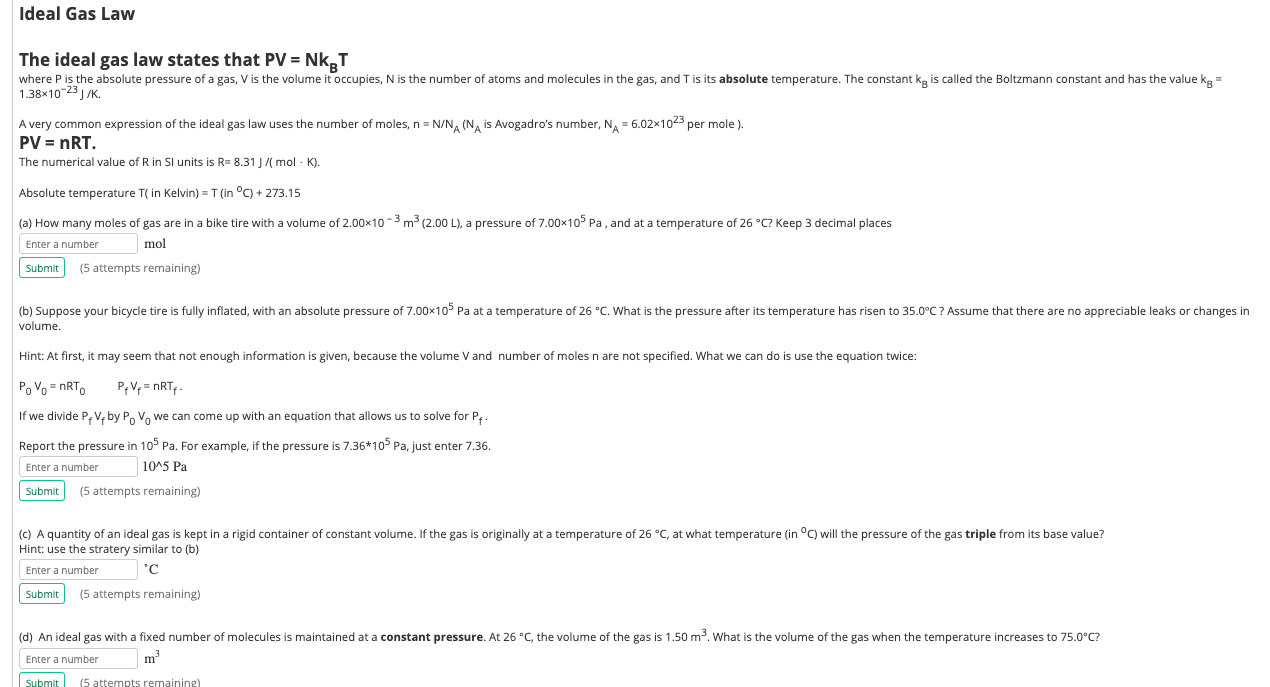Please answer all parts of the question: a,b,c,d Ideal Gas Law The ideal gas law states that PV = Nk T where P is the absolute pressure of a gas, V is the volume it occupies, N is the number of atoms and molecules in the gas, and T is its absolute temperature. The constant kg is called the Boltzmann constant and has the value kg = 1.38x10-29 J/K. A very common expression of the ideal gas law uses the...

• ### Bicycle We has a pressure of 7.00-105 N/m2 af a tem HW 10 - Thermal Physics...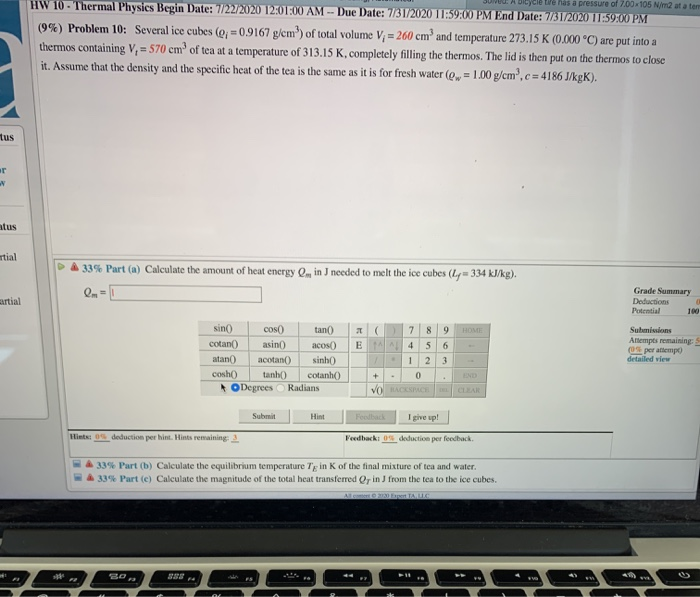Bicycle We has a pressure of 7.00-105 N/m2 af a tem HW 10 - Thermal Physics Begin Date: 7/22/2020 12:01:00 AM - Due Date: 7/31/2020 11:59:00 PM End Date: 7/31/2020 11:59:00 PM (9%) Problem 10: Several ice cubes (q;= 0.9167 g/cm) of total volume V; = 260 cm and temperature 273.15 K (0.000 °C) are put into a thermos containing V,= 570 em of tea at a temperature of 313.15 K, completely filling the thermos. The lid is then put...